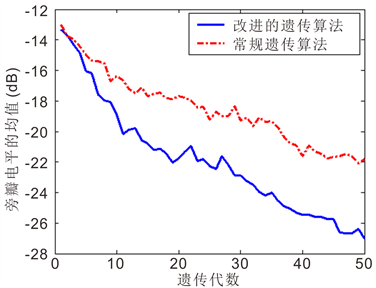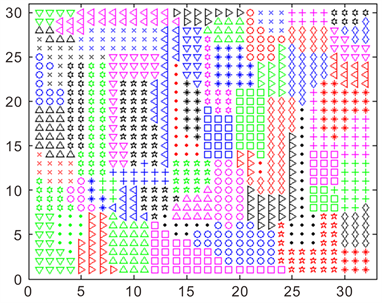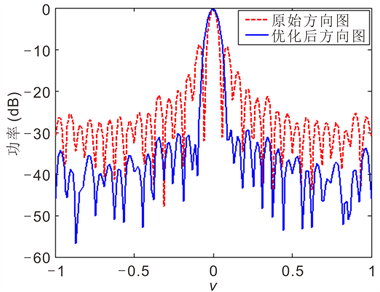# 基于改进遗传算法的相控阵雷达最优子阵划分Optimal Subarray Partition of Phased Array Radar Based on Improved Genetic Algorithm

DOI: 10.12677/JA.2018.71001, PDF, HTML, XML, 下载: 590  浏览: 1,620

Abstract: Subarray structure is usually adopted by phased array radar, and has a significant impact on the performance of the system. The optimal subarray division is the important task with great practical significance. The genetic algorithm was used to optimize the subarray to get optimal sidelobe performance. The objective function of the genetic algorithm and the encoding and decoding method of the subarray structure were given. The improved genetic algorithm based on adaptive crossover operator was proposed, which has the characteristics of adaptive cross combination and more optimized offspring population, and so the convergence speed and operational efficiency were greatly improved. Simulation results show the effectiveness of the proposed method.

1. 引言

2. 基于遗传算法的最优子阵划分

2.1. 遗传算法

2.2. 目标函数

$u=\mathrm{sin}\theta \mathrm{cos}\phi$$v=\mathrm{sin}\theta \mathrm{sin}\phi$${u}_{\text{0}}=\mathrm{sin}{\theta }_{\text{0}}\mathrm{cos}{\phi }_{\text{0}}$${v}_{\text{0}}=\mathrm{sin}{\theta }_{\text{0}}\mathrm{sin}{\phi }_{\text{0}}$ ，并设

${P}_{0}=\text{diag}{\left\{{g}_{m}\mathrm{exp}\left[-\text{j}2\text{π}\left({x}_{m}{u}_{0}+{y}_{m}{v}_{0}\right)/\lambda \right]\right\}}_{m=1,\cdots ,M}$ (1)

$T={P}_{0}{T}_{0}$ (2)

${a}_{\text{sub}}\left({\theta }_{0},{\phi }_{0}\right)={T}^{\text{H}}a\left({\theta }_{0},{\phi }_{0}\right)$ (3)

${w}_{\text{sub}}$ 为子阵级加权向量(用于进行数字处理)，则以 $\left(u,v\right)$ 为自变量的阵列方向性函数为

$f\left(u,v\right)={\left({w}_{\text{sub}}\right)}^{\text{H}}\left[{a}_{\text{sub}}\left(u,v\right)\circ {a}_{\text{sub}}\left(-{u}_{0},-{v}_{0}\right)\right]$ (4)

2.3. 子阵结构的编解码方法

2.4. 子阵划分的约束条件

1) 解码后所有阵元均位于阵列的有效范围内

2) 同一子阵中所有的阵元相邻

3) 各子阵为非重叠子阵

4) 阵列为满布阵Figure 1. Schematic diagram of chromosome decoding

3. 基于自适应交叉算子的改进遗传算法

${{P}^{\prime }}_{c}=\frac{{f}_{\text{Ave}}}{{f}_{\text{Opt}}}$ (5)

4. 子阵划分流程

1) 随机产生中心染色体及周围染色体；

2) 对中心染色体和周围染色体解码，还原子阵结构；

3) 根据解码结果，判断是否满足约束条件，并修正染色体中的非法基因；即使同一子阵中所有阵元相邻，各子阵为非重叠子阵且阵列为满布阵；

4) 计算由解码得到的子阵结构所相应的方向图的旁瓣电平及适应度值；

5) 求出旁瓣电平的最优值及平均值，计算自适应交叉算子；

6) 分别对中心染色体和周围染色体进行选择、交叉及变异操作，以产生新的染色体；

7) 判断是否满足迭代次数，是则终止，否则返回2。

5. 仿真结果

5.1. 仿真条件

5.2. 改进遗传算法的收敛性能Figure 2. Sub array optimization flow chart based on Improved Genetic AlgorithmFigure 3. Comparison of convergence performance between the two methods

5.3. 方向图

5.4. 子阵划分结果Figure 4. Subarray optimization resultsFigure 5. Directional map